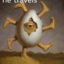MathematicsOpenStudy (anonymous):

The Endpoints of LF are L(-2,2) and F(3,1). The endpoints of JR are J(1,-1) and R(2,-3). What is the approximate difference in the lengths of the two segments?OpenStudy (anonymous):

Could someone help with how to do not just the answer? Thxjimthompson5910 (jim_thompson5910):

Distance from L to F (ie length of LF) d = sqrt((x2-x1)^2+(y2-y1)^2) d = sqrt((3-(-2))^2+(1-2)^2) d = sqrt((5)^2+(-1)^2) d = sqrt(25+1) d = sqrt(26) So the exact distance between points L and F is sqrt(26) units. Using a calculator, this approximates to 5.099 Use the formula above to calculate the distance between J and R. Once you have this distance, simply subtract.OpenStudy (anonymous):

Thanks a bunch!

Latest QuestionsShelbiLW: A 3.0 m uniform beam of mass 15 kg is pivoted 1.0 m from the end as shown below. A 35 kg child sits 0.
4 hours ago 0 Replies 0 Medalsryleighmullins22: Answer the question below using the following amortization table. Month Principal Paid Interest Paid Balance 21 \$654.
4 hours ago 0 Replies 0 MedalsTonycoolkid21: help
4 hours ago 8 Replies 0 MedalsTonycoolkid21: help
5 hours ago 15 Replies 3 MedalsRx1nyDxyz: Help If the supply of a product increases, then the price decreases*** the price
6 hours ago 21 Replies 1 Medalshototodorokii: (not from a test/quiz)Nomenclature is governed by certain universal rules. Which
7 hours ago 0 Replies 0 Medalscrispyrat: A guide to sqrt and cbrt
5 hours ago 21 Replies 3 MedalsxXQuintonXx: help please(ss below)
7 hours ago 5 Replies 1 Medalajfknkjfa: Prove that the two circles shown below are similar. (10 points) Circle C is shown with a center at negative 3, 1 and a radius of 4.
7 hours ago 3 Replies 1 Medalrxcklesskaisher: idk wdh dis is
7 hours ago 2 Replies 0 Medals